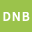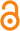Please use this identifier to cite or link to this item: `http://dx.doi.org/10.25673/108915`
 Title: On the formulas for Pi(​x) and Psi(x) of Riemann and von Mangoldt Author(s): Kunik, MatthiasIssue Date: 2023 Extent: 1 Online-Ressource (42 Seiten) Type: Preprint Language: English Publisher: Universitätsbibliothek, Magdeburg URN: urn:nbn:de:gbv:ma9:1-1981185920-1108703 Subjects: Explicit formulasDistribution of prime numbersRiemann zeta functionFourier analysis Abstract: Using the Mellin transform and the complex exponential integral we derive various representation formulas for the factors of the entire functions in Hadamards product theorem. The application of these results on Riemann’s zeta function leads to a new derivation of Rie- mann’s prime number formula for Pi(x). We will thereby present a correct version of this formula, which is given in a wrong way in the literature. Using the nontrivial zeros of the Zeta function we also obtain explicit formulas for regularizations of von Mangoldt’s function Psi(x). These regularizations are based on cardinal B-splines and Gaussian integration kernels, which are related by the Central Limit Theorem. Our results will then be generalized to a windowed Mellin or Fourier transform with a Gaussian window function. URI: https://opendata.uni-halle.de//handle/1981185920/110870http://dx.doi.org/10.25673/108915 Open Access:Open access publication License:(CC BY-SA 4.0) Creative Commons Attribution ShareAlike 4.0 Appears in Collections: Fakultät für Mathematik (OA)